• #@tf.function def cross_layer(x0, xl): embed_dim = xl.shape[-1] w = tf.Variable(tf.random.truncated_normal(shape=(embed_dim,), stddev=0.01),trainable=True) b = tf.Variable(tf.zeros(shape=(embed_dim...shell
• ASON网络是现在世界上光传送网络研究的热点，它是原有光传送网络的一次重大演进，具有许多原有光传送网络不可比拟的优点。目前ASON网络设备在国际上的现网中已经进入了规模应用，并将逐步部署在我国的光传送网络中。
• 伴随着医院信息系统的建设，如何保障信息系统的安全性逐渐被各大...早在2002年发布的《医院信息系统基本功能规范》中，就提出了HIS系统的高效安全原则，提出机房、网络和硬件系统必须按照国家相关规范和标准进行建设。
• ## DCN

千次阅读 2018-08-12 18:30:17
一个DCN模型从嵌入和堆积层开始，接着是一个交叉网络和一个与之平行的深度网络，之后是最后的组合层，它结合了两个网络的输出。完整的网络模型如图：   嵌入和堆叠层 我们考虑具有离散和连续特征的输入数据...

Deep&Cross Network模型我们下面将简称DCN模型：
一个DCN模型从嵌入和堆积层开始，接着是一个交叉网络和一个与之平行的深度网络，之后是最后的组合层，它结合了两个网络的输出。完整的网络模型如图：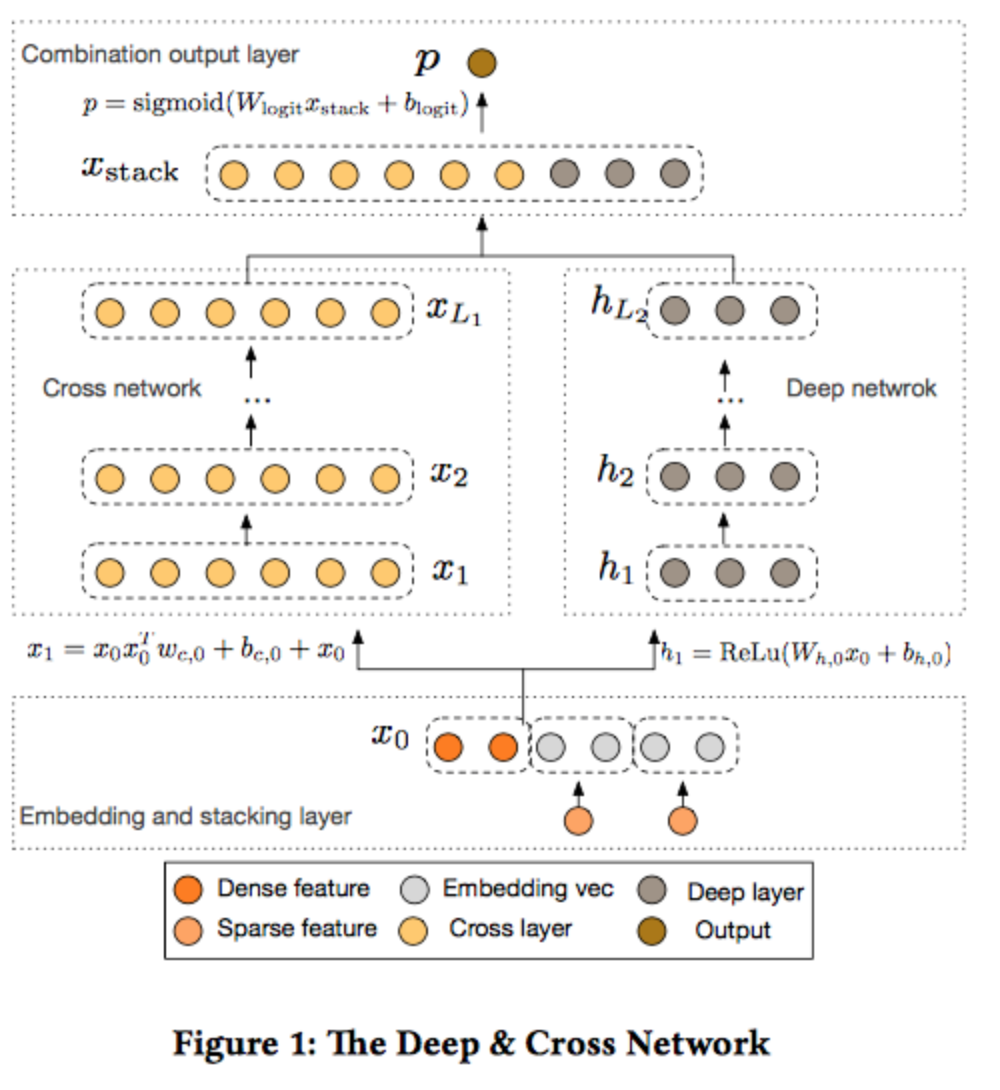嵌入和堆叠层 我们考虑具有离散和连续特征的输入数据。在网络规模推荐系统中，如CTR预测，输入主要是分类特征，如“country=usa”。这些特征通常是编码为独热向量如“[ 0,1,0 ]”；然而，这往往导致过度的高维特征空间大的词汇。

为了减少维数，我们采用嵌入过程将这些离散特征转换成实数值的稠密向量（通常称为嵌入向量）：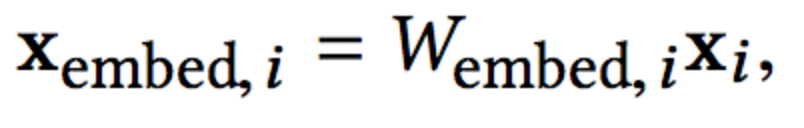然后，我们将嵌入向量与连续特征向量叠加起来形成一个向量：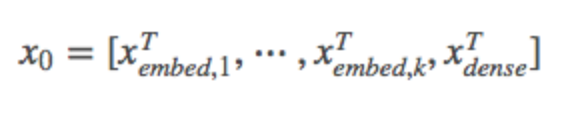Cross Network 交叉网络的核心思想是以有效的方式应用显式特征交叉。交叉网络由交叉层组成，每个层具有以下公式：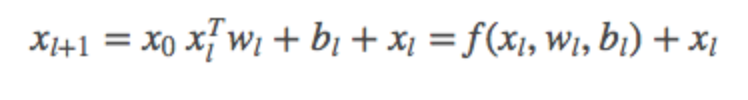总结 DCN能够有效地捕获有限度的有效特征的相互作用，学会高度非线性的相互作用，不需要人工特征工程或遍历搜索，并具有较低的计算成本。 论文的主要贡献包括：

1）提出了一种新的交叉网络，在每个层上明确地应用特征交叉，有效地学习有界度的预测交叉特征，并且不需要手工特征工程或穷举搜索。 2）跨网络简单而有效。通过设计，各层的多项式级数最高，并由层深度决定。网络由所有的交叉项组成，它们的系数各不相同。 3）跨网络内存高效，易于实现。 4）实验结果表明，交叉网络（DCN）在LogLoss上与DNN相比少了近一个量级的参数量。
展开全文• 全光网状网络DCN布局和可生存的云服务提供的联合设计研究论文
• 华为公司Quidway:registered:AR系列路由器，支持ISDN/X25/FR/ATM/DDN等多种接入方式，也能提供从E1到1000M的高速传输链路，充分满足电信公司DCN网络对于接入路由器和核心路由器可用性方面的要求。
• 配置无线网络，首先需要启用DCWS的无线功能，将AP认证模式设置为不认证，指定无线模块的地址即AP所在VLAN的网关地址，network1为无线网络1（共可以配置16个无线网络即network1-16），这一块需要配置无线网络的名称...
根据技能大赛无线AP题目的配置要求，DCWS无线交换机又称AC无线控制器，需要配置多个VLAN，在此总结了基本配置的三个部分。第一部分：1.配置VLAN和接口IP，VLAN66用于连接三层交换机DCRS，VLAN110用于连接无线AP，VLAN111和VLAN222为用户数据VLAN。2.将接入AP的接口配置为Trunk，无线AP接在DCWS的23号口上，并配置本质VLAN110。第二部分：开启DHCP功能，配置DHCP服务，配置AP所在VLAN的DHCP服务时应静态指定AC的IP地址（0101是固定值，后边的是IP地址的16进制表现形式，最多跟4个AC地址），其他VLAN的DHCP服务配置可根据题目要求来进行配置。第三部分：配置无线网络，首先需要启用DCWS的无线功能，将AP认证模式设置为不认证，指定无线模块的地址即AP所在VLAN的网关地址，network1为无线网络1（共可以配置16个无线网络即network1-16），这一块需要配置无线网络的名称（SSID），无线网络的加密方式以及无线密码，并配置无线网络所属的VLAN。展开全文• 用户通常遍布全球各地，需要通过日益多样化的移动设备接入网络。组成应用的不同单元可能在不同服务器上运行，而所有这些服务器都必须互相通信，检索需要的信息并以根据用户需求定制的形式提供给用户。很高的带宽和...
• DCN和cascade在DOTA数据集上训练的代码、模型、精度误差曲线等全部数据
• ## DCN解读

万次阅读 2019-08-30 17:38:03
DCN论文的贡献是提出Deformable Conv。 论文创新： 1、Deformable Conv 2、Deformable ROI pooling 背景 深度学习对图像处理往往需要大量的数据集训练，是因为我们需要让网络学习到所有可能存在的情况，所以我们采用...
DCN论文的贡献是提出Deformable Conv。
论文创新：
1、Deformable Conv
2、Deformable ROI pooling
背景
深度学习对图像处理往往需要大量的数据集训练，是因为我们需要让网络学习到所有可能存在的情况，所以我们采用了数据增强的方法，如旋转、左右移、上下移、裁剪等操作。
但是针对图像目标尺度、姿态、角度、局部的变形等情况，基于经典卷积固定的几何结构，我们并不能完全模拟出来。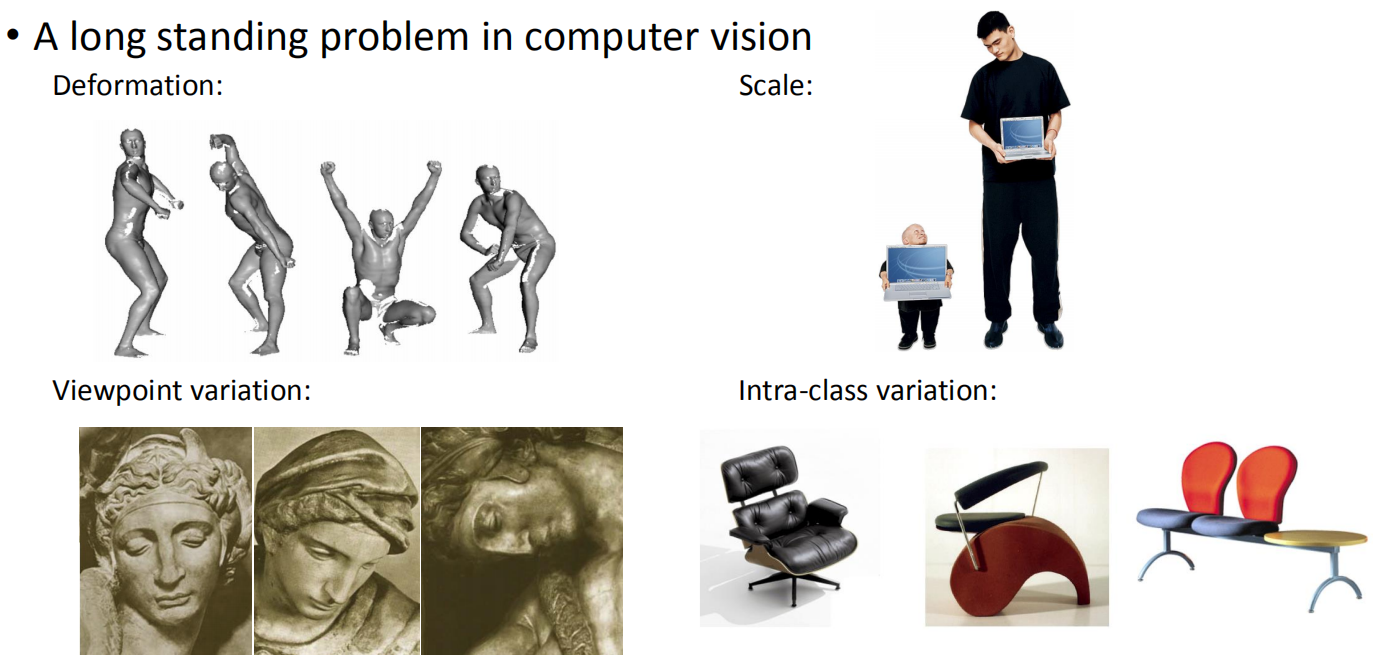方法
于是，作者提出Deformable，对于3×3卷积核，经典卷积是第一幅图那样简单，紧密排列的样子；第三幅图是针对尺度和纵横比，改变之后的卷积核，类似与空洞卷积；第四幅图是针对图像旋转的卷积核；而第二幅是作者提出的，针对任意的图像改变，网络会学习到卷积核的一个偏移量，使网络可以适应目标不同的变化。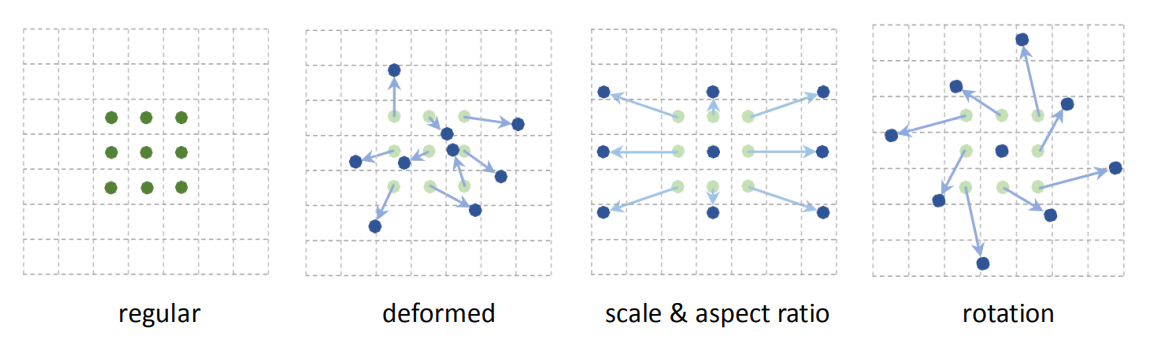Deformable Conv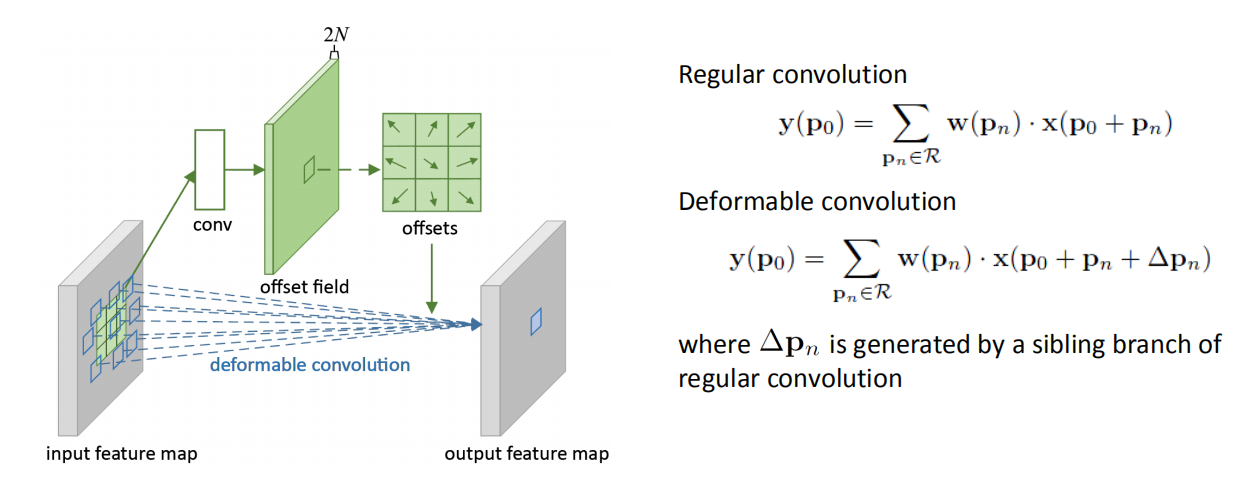w(pn)是网络学习的参数（权重），即卷积核；x(p0+pn)是像素p0+pn的像素值，如下图，我们使用3×3的卷积核，pn是p0附近的8个坐标。
Δpn是基于经典卷积的偏移量，是需要输入数据通过网络学习而得的。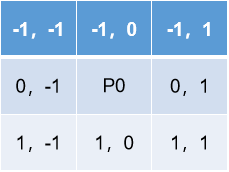Deformable ROI pooling
看这个之前需要先了解一下Fast RCNN的ROI pooling，大致就是将ROI映射后的特征图分成几个小窗口，然后对每个小窗口做max pooling。可以看下我的Fast RCNN论文解读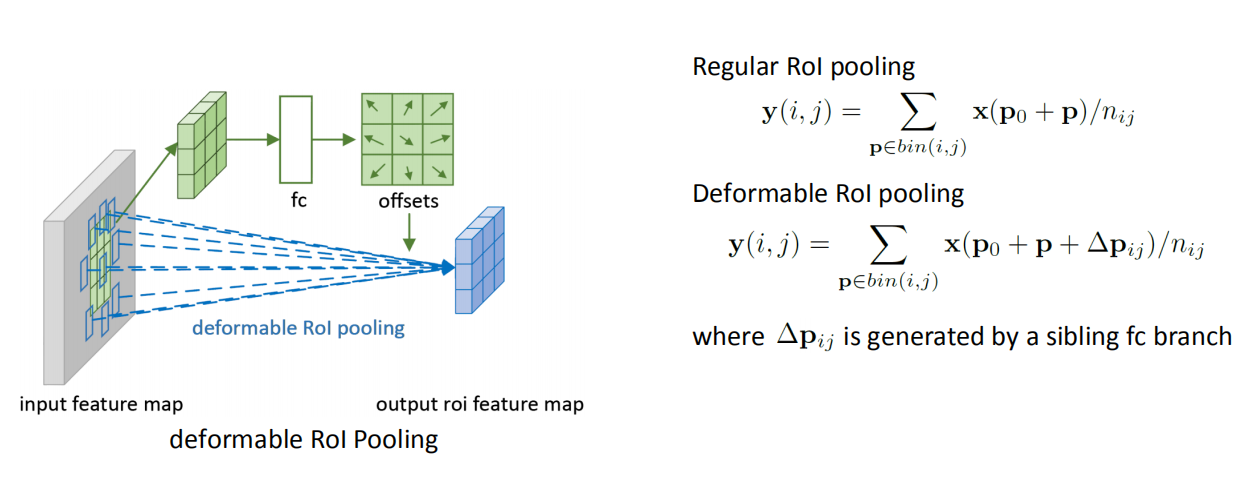Fast RCNN取得是max pooling，而本文中是取得mean pooling。这里也是通过学习它的偏移量，使ROI映射的小窗口更接近于真实的目标。
实例
Deformable Conv
这里每个图像针对采样位置（其中一个小绿点），使用3个激活单元（b图是显示的2个激活单元），采用3×3的deformable卷积核，所以（3×3）^3=729个小红点。
下图中左侧是分布的背景，中间是小目标分布，右图是大目标分布。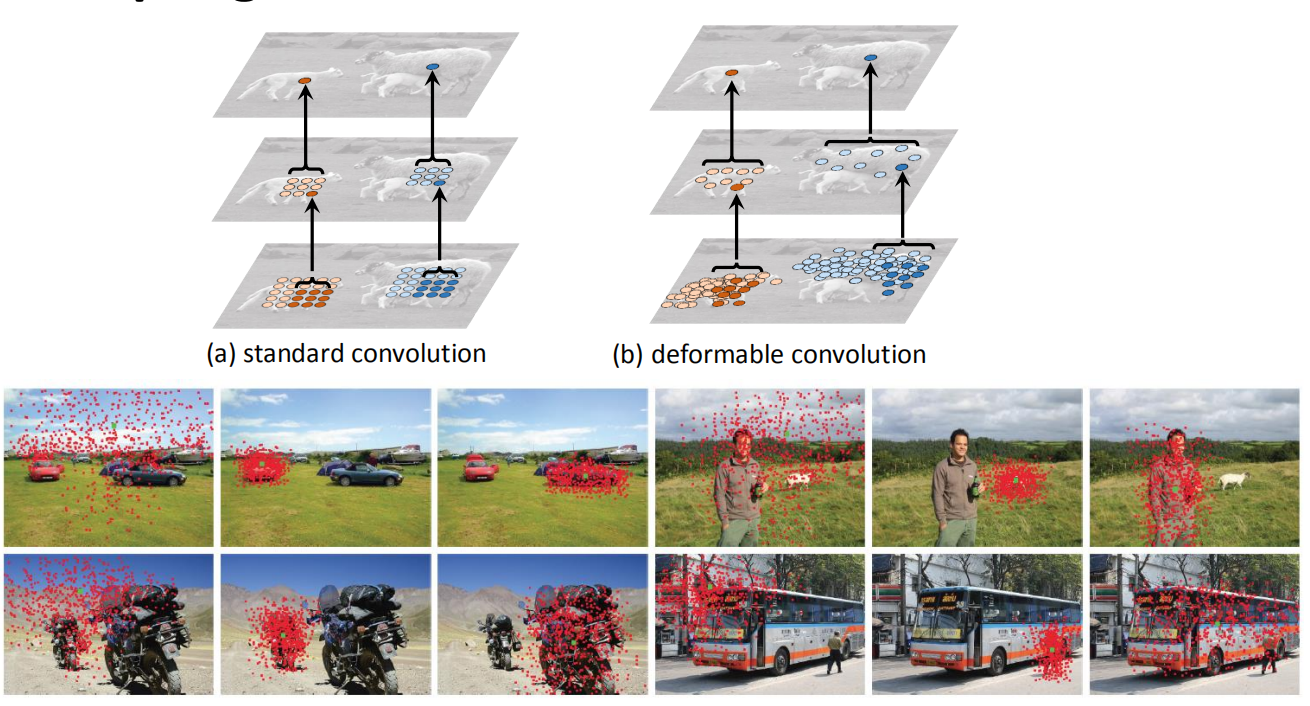Deformable ROI pooling
这里将ROI映射分成3×3=9个小窗口，图中可以很容易理解，这9个小窗口都更偏向于目标。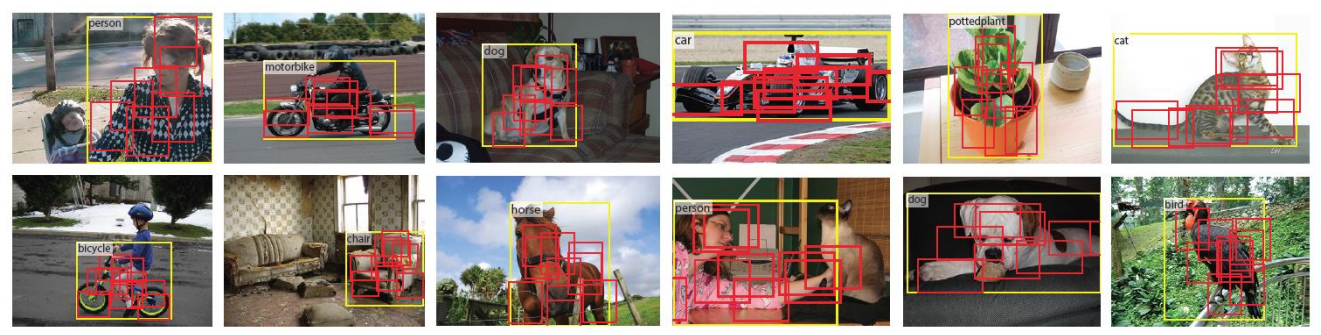展开全文• Deep Cross Network (深度交叉网络, DCN) 介绍与代码分析 文章目录Deep Cross Network (深度交叉网络, DCN) 介绍与代码分析文章信息主要内容Deep Cross Network特征输入Cross NetworkDeep NetworkCombination Layer...
Deep Cross Network (深度交叉网络, DCN) 介绍与代码分析
文章目录Deep Cross Network (深度交叉网络, DCN) 介绍与代码分析广而告之文章信息主要内容Deep Cross Network特征输入Cross NetworkDeep NetworkCombination Layer总结参考资料
发现看完 Paper 和源码后如果不做点笔记, 一段时间过后, 甚至记不起曾经看过相应的 Paper, 年纪大了啊 😂😂😂, 所以得养成好习惯. 限于水平, 笔记记录下 Paper 的要点即可. 说回正题, 这是 Stanford & Google 于  2017 年发表于 KDD 的工作.

Ruoxi Wang, Bin Fu, Gang Fu, and Mingliang Wang. 2017. Deep & Cross Networkfor Ad Click Predictions. In Proceedings of the ADKDD’17. ACM, 12.

后面的代码分析采用 DeepCTR-Torch 的 PyTorch 实现.
广而告之
可以在微信中搜索 “珍妮的算法之路” 或者 “world4458” 关注我的微信公众号；另外可以看看知乎专栏 PoorMemory-机器学习, 以后文章也会发在知乎专栏中；
文章信息
文章地址: Deep & Cross Networkfor Ad Click Predictions
发表时间: KDD, 2017
代码实现: https://github.com/shenweichen/DeepCTR-Torch/blob/master/deepctr_torch/models/dcn.py
主要内容
传统的 CTR 模型为了增强非线性表达能力, 需要构造特征组合, 虽然这些特征含义明确、可解释性强, 但通常需要大量的特征工程, 耗时耗力. 另一方面, DNN  模型具有强大的学习能力, 可以自动获取高阶的非线性特征组合, 然而这些特征通常是隐式的,  含义难以解释. 本文的亮点在于提出了一个 Cross Network, 可以显式并且自动地获取交叉特征; 此外, Cross Network 相比 DNN 显得更为轻量级,因此在表达能力上比 DNN 会逊色一些, 本文通过联合训练 Cross Network 与 DNN 来同时发挥二者的优势.
Deep Cross Network
下图即为本文提出的 DCN 完整模型: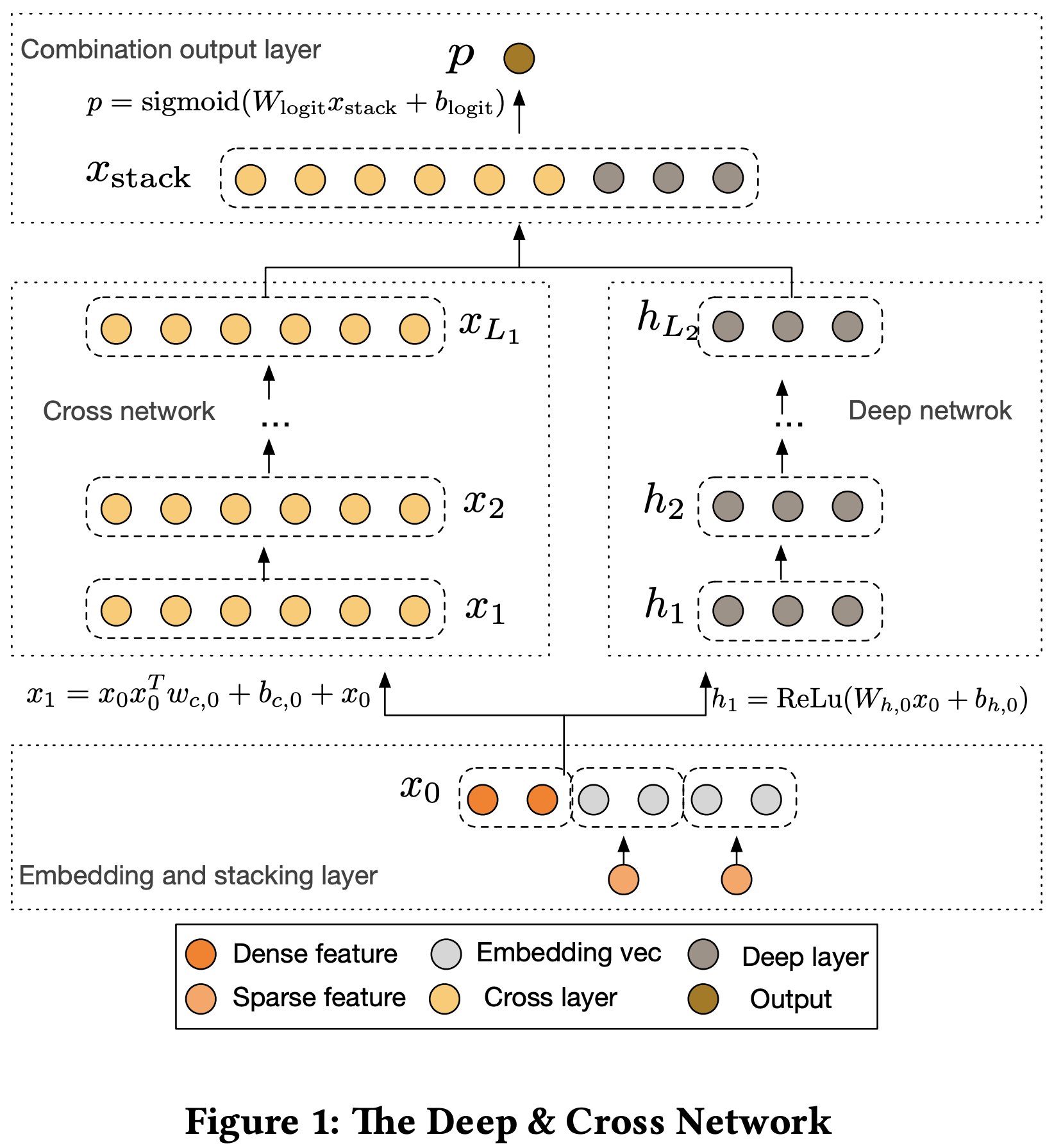主要由三部分构成:

底层的 Embedding and Stacking Layer
中间层并行的 Cross Network 以及 Deep Network
输出层的 Combination output Layer

特征输入
对于原始的输入特征, 可能包括离散的类别特征 (Categorical Features) 和连续的稠密特征 (Dense Features). 通常我们会将类别特征编码为 OneHot 的形式, 但会导致对应的特征维度较高且稀疏, 因此我们还会引入一个 Embedding 层将类别特征映射为低维的稠密特征:
$x_{emb, i} = W_{emb, i} x_i$
之后和规范化的连续特征进行 Concatenation 后, 再喂入到下一层网络中:
$x_0 = [x^T_{emb, 1}, x^T_{emb, 2}, \ldots, x^T_{emb, k}, x^T_{\bm{dense}}]$
Cross Network
先看图, 再看公式化表达: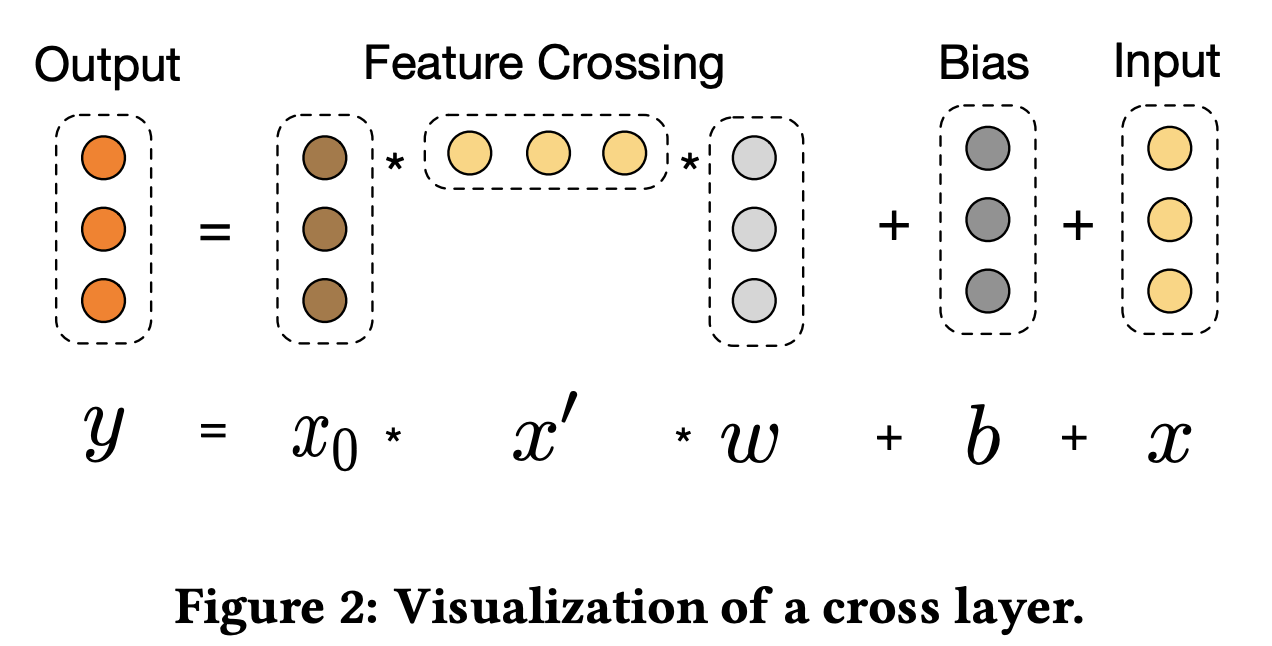Cross Network 是本文的核心 idea, 它被用来显式并且高效地对交叉特征进行学习. 它由多个 Crossing Layer 构成, 每一层使用下式表示:
$x_{l + 1} = x_0 x^T_l w_l + b_l + x_l = f(x_l, w_l, b_l) + x_l$
其中 $x_{l}, x_{l + 1}\in\mathbb{R}^n$ 分别为第 $l$ 层以及第 $l + 1$ 层的输出特征; $w_l, b_l\in\mathbb{R}^n$ 为第 $l$ 层的权重和偏置; 注意到权重 $\bm{w}$ 为向量而非矩阵. 此外, Residual Learning 的思路也被引入, 可以有效避免梯度消失的问题, 并可以帮助构建更深的网络, 增强网络的表达能力. Cross Network 特殊的结构可以让特征的阶数(degree)随着网络的深度增加而增长. 比如一个层数为 $l$ 的 Cross Network, 其 highest polynomial degree 为 $l + 1$.
由于每一层 cross layer 的权重 $w$ 和偏置 $b$ 都是向量, 假设维度均为 $d$, 那么层数为 $L_c$ 的 Cross Network 的参数总量为 $d\times L_c\times 2$. 由于 Cross Network 的参数数量相对较少, 这限制了模型的表达能力, 因此需要配合 Deep Network 一起使用.
下面看看 DeepCTR-Torch 对 CrossNet 的 PyTorch 实现:
class CrossNet(nn.Module):
"""The Cross Network part of Deep&Cross Network model,
which leans both low and high degree cross feature.
Input shape
- 2D tensor with shape: (batch_size, units).
Output shape
- 2D tensor with shape: (batch_size, units).
Arguments
- **in_features** : Positive integer, dimensionality of input features.
- **input_feature_num**: Positive integer, shape(Input tensor)[-1]
- **layer_num**: Positive integer, the cross layer number
- **l2_reg**: float between 0 and 1. L2 regularizer strength applied to the kernel weights matrix
- **seed**: A Python integer to use as random seed.
References
- [Wang R, Fu B, Fu G, et al. Deep & cross network for ad click predictions[C]//Proceedings of the ADKDD'17. ACM, 2017: 12.](https://arxiv.org/abs/1708.05123)
"""

def __init__(self, in_features, layer_num=2, seed=1024, device='cpu'):
super(CrossNet, self).__init__()
self.layer_num = layer_num
self.kernels = torch.nn.ParameterList(
[nn.Parameter(nn.init.xavier_normal_(torch.empty(in_features, 1))) for i in range(self.layer_num)])
self.bias = torch.nn.ParameterList(
[nn.Parameter(nn.init.zeros_(torch.empty(in_features, 1))) for i in range(self.layer_num)])
self.to(device)

def forward(self, inputs):
x_0 = inputs.unsqueeze(2)
x_l = x_0
for i in range(self.layer_num):
xl_w = torch.tensordot(x_l, self.kernels[i], dims=(, ))
dot_ = torch.matmul(x_0, xl_w)
x_l = dot_ + self.bias[i] + x_l
x_l = torch.squeeze(x_l, dim=2)
return x_l

其中 layer_num 表示 Cross Network 的层数, 由于权重和 Bias 均为向量, 代码中使用 PyTorch 中的 Parameter 来表示, 每一层权重/Bias 的大小为 [in_features, 1], 为了方便我们设 in_channels = d.
在 forward 方法中实现 Cross Network 的前向传播逻辑. 其中 inputs 的大小为 [B, d], 其中 B 表示一个 Batch 的大小, d 表示每个样本的维度, 即输入特征的维度.
x_0 = inputs.unsqueeze(2)

这一步对 inputs 的 dim=2 进行扩展, 此时 x0 的 Shape 为 [B, d, 1]. 之后在 for 循环中, 实现公式
$x_{l + 1} = x_0 x^T_l w_l + b_l + x_l$
的效果. 第一步先完成 $x^T_l w_l$, 即:
xl_w = torch.tensordot(x_l, self.kernels[i], dims=(, ))

这里需要了解下 torch.tensordot, 它将 x_l : [B, d, 1] 的 dim=1 这个维度的数据, 即 d 这个维度的数据, 也即特征, 和第 $l$ 层的权重 kernels[i] : [d, 1] 的 dim=0 这个维度的数据, 也就是权重, 进行 element-wise 相乘并求和. 最终的效果就是 Shape 为 [B, d, 1] 的特征和 Shape 为 [d, 1] 的权重进行 tensordot 后得到 Shape 为 [B, 1, 1] 的结果.
第二步进行 $x_0 x^T_l w_l$, 由于前一步得到了 $x^T_l w_l$, 代码中表示为 xl_w, 那么这一步的结果为:
dot_ = torch.matmul(x_0, xl_w)

这时候得到的 dot_ Shape 和 x_0 相同, 均为 [B, d, 1]
最后一步是加上偏置以及输入自身:
x_l = dot_ + self.bias[i] + x_l

经过 layer_num 次循环后, 也即特征经过了 $l$ 层后, 代码中的 x_l  Shape 为 [B, d, 1], 因此还需进行最后一步:
x_l = torch.squeeze(x_l, dim=2)

得到大小为 [B, d] 的输入结果.
Deep Network
这个不多说, 里面可能会用到 Dropout, BN 之类的, 但作者在论文 4.2 节的实现细节中说没有发现 Dropout 或者 L2 正则化有效.
Deep Network 的公式化为:
$h_{l + 1} = f(W_l h_l + b_l)$
Combination Layer
最后的输出层, 需要先将 Cross Network 与 Deep Network 的结果进行 Concatenation, 输入到 LR 模型 (对于 LR 模型, 可以详见文章 逻辑回归模型 Logistic Regression , 推导特别详细, 我都很感动 😂😂😂), 从而得到预测概率.
$p = \sigma\left([x^T_{L_1}, h^T_{L_2}]w_{logits}\right), \quad \sigma(x) = \frac{1}{1 + e^{-x}}$
损失函数为 LR 模型的 Negative Log Likelihood, 再加上 L2 正则项:
$\text{loss} = -\frac{1}{N}\sum_{i=1}^{N}y_i\log(p_i) + (1 - y_i)\log(1 - p_i) + \lambda\sum_{l}\Vert \bm{w}_l\Vert^2$
使用 Adam 算法进行优化.
总结
文章介绍的 Cross Network 可以用于高效地对高阶组合特征进行显式学习, 组合特征的多项式阶数会随着网络深度的增加而增加. Cross Network 每一层的权重均为向量, 结构相对较简单, 参数相比 DNN 显著减少, 这也限制了模型的表达能力, 因此需要联合 DNN 训练, 两者优势互补.
参考资料

Deep & Cross Networkfor Ad Click Predictions 论文资料
DeepCTR-Torch 实现了经典的各种 CTR 模型, 非常推荐看源码学习.
(读论文) 推荐系统之ctr预估-DCN模型解析 深入读论文系列


展开全文机器学习 ctr 深度学习
• ## DCN V2

2020-05-22 14:53:58
分析DCN Spatial Support Visualization Effective receptive fields 理论上现在很多深层网络最后feature上每个位置的视野域都是铺满全图的，但是实际视野域中每个点对响应的不同，有效视野域即为输入扰动后实际能...深度学习
• 概述 该系列主要是复现一些经典的网络结构与顶会论文的网络结构，我一开始看论文，以为看到网络结构和了解结构原理后，就完全学到了这篇论文的精髓，谁知，等到自己想要用这个网络结构时，无法打通理解与代码复现的...推荐系统
• 神州数码路由器NAT 配置教程。DCN-ROUTE-NAT-网络地址转换
• 具体的DCN模型介绍看上篇的介绍 2.代码复现 该部分主要是按照网络结构图，用代码的方式实现。在代码实现的过程中，我们需要紧紧结合数学公式体会其中的含义以及如何用代码来实现这些数学公式。 该模型主要分为三...
• 使用华为ENSP模拟器验证DCN穿越第三方二层网络，附上幕布笔记链接 https://share.mubu.com/doc/6Mx9eTqKPin
• 本文所重点叙述的IP RAN是指以IP/MPLS动态协议及关键技术为基础，满足基站回传承载需求的一种二层三层技术结合的解决方案。以下分析IP RAN网络的关键需求及相关技术，需要的朋友可以参考下
• 深度特征交叉网络 之前常常研究CNN的网络结构，却不知道多层感知机（MLP）的设计也有很多讲究。可以设计出很多不同的网络。 对于传统的分类算法来说，输入数据通常是一个一维的向量，向量中的每个值都是一个特征，这...
• 本文件其实和msra_resnet.py类似，进入博文，这里只把两者的不同说下，不同的部分有以下几处，总体感觉作者在这里添加DCN模块很牵强（仅个人吐槽），可能对反卷积有帮助吧： # 反卷积操作的通道不同 # used for ......